Next: 4.3 Sparse Field Implementation Up: 4.2 Sequential Data Access Previous: 4.2.1 Basic Iterator

## 4.2.2 Offset Iterator

If access to neighbor grid points is needed, additional iterators are used which are moved simultaneously with a central basic iterator over the H-RLE data structure [44,84]. Each additional iterator has a predefined offset relative to the central iterator. For example, to enable the calculation of first order differences in two dimensions four additional iterators are necessary with offset index vectors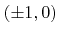and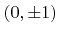.

As described earlier the H-RLE data structure is able to store the LS values of a finite set of points of a grid with infinite extensions. However, sometimes it is desireable to limit the simulation domain by using periodic or reflective boundary conditions. In topography simulations, such boundaries are usually used for lateral extensions. (Infinite boundaries are also implemented as reflective boundaries, for which the corresponding minimum and maximum grid indices are set to the limits of the used integral data type.)

Boundary conditions must be incorporated while traversing an offset iterator over the H-RLE data structure. The movement is not as continuous as for the basic iterator, because, due to the boundary conditions, leaps within the data structure may be necessary to reach the next position. At reflective boundaries the moving direction of an offset iterator may also be reversed.

To enhance the usability of iterators an offset iterator has been implemented which provides the same functionality as the basic iterator and which automatically incorporates the applied boundary conditions. It is initialized with a certain offset index vector. The behavior of an offset iterator amounts to a basic iterator moving over the H-RLE data structure, obtained by shifting all segments of the H-RLE data structure according to the (reversed) offset with consideration of the boundary conditions.

Figure 4.3 exemplifies the behavior of offset iterators. Figure 4.3a shows a small surface which is resolved on a simulation domain of size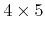, with reflective and periodic boundary conditions along the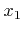- and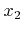-direction, respectively. Storing the LS values of defined grid points using the H-RLE data structure leads to the segmentation as given in Figure 4.3b. Figure 4.3c and Figure 4.3d show the segmentation of the H-RLE data structure as seen for iterators with offsets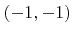and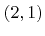, respectively.

The-iterator gives access to the-neighbors of all grid points with indices in the range given by the start and end index vectors returned by the offset iterator. For example, the fourth position of the-iterator refers to the positive undefined run with segment number 2. The minimum and maximum index vector are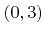and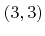, respectively, which means that the referenced segment contains the-neighbors for the grid points,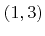,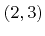, and. A look at Figure 4.3b confirms that for these grid points the-neighbors belong to the positive undefined run with number 2. During a complete traversal of the- and the-iterator the segments of the H-RLE data structure are passed in the order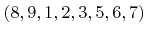and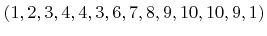, respectively. For reflective boundaries it may not be necessary for all segments to be visited by an offset iterator. For example, the-iterator never stops at segment 4.

A traversal over the entire H-RLE data structure can be performed with linear complexity for all offset iterators, independent of the offset index vector. In most cases, the next segment can be reached in constant time by stepping forward, similar to the basic iterator. Alternatively, in the case of reflective boundary conditions backward steps can be necessary, as is the case for the movement of the-offset iterator from point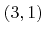to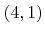(see Figure 4.3d). If the next iterator position is neither the next nor the previous segment, a random access operation is necessary. This is the case, when the next iterator position is on another-grid line (parallel to the-direction) and the first position of the offset iterator must be found there. Due to the offset the first segment is not necessarily the first position.

A random access operation requires a binary search. In order to estimate the additional costs for the binary searches during a complete traversal over the H-RLE data structure all-grid lines, to which at least one defined grid point belongs, are considered. Let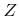be the number of these grid lines. The distribution of defined grid points over thesegrid lines is given by the positive numbers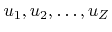with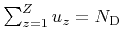.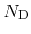is the total number of defined grid points. Iterators will only traverse these grid lines. In order to find the start position on the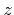-th grid line a binary search is necessary which has a worst case logarithmic complexity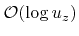. Hence, the total costs during a complete traversal over the H-RLE data structure are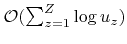which can be further estimated as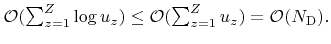(4.7)

Therefore, the total additional costs due to the binary searches for the worst case scenario are of order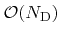, which proves the linear complexity for a complete traversal of offset iterators.

Several offset iterators can be grouped and moved simultaneously over the data structure to enable neighbor access to grid points. As an example, an iterator for first order finite differences in two dimensions can be built from 5 iterators with offsets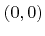,, and. The common set of grid points of all iterators is given by the maximum of all their minimum index vectors and the minimum of all their maximum index vectors. The current iterator positions only apply for grid points within this range. To go to the next grid points the common range must be moved forward. This is performed by advancing only those iterators for which their maximum index vector is equal to the upper bound of the common range. In this way an iteration over the H-RLE data structure can be carried out in linear time. However, by nature, the costs are directly proportional to the number of iterators in the group. Due to the inherent incorporation of boundary conditions arbitrary stencils of iterators can be easily formed. This allows a simple implementation of algorithms for the H-RLE data structure.Next: 4.3 Sparse Field Implementation Up: 4.2 Sequential Data Access Previous: 4.2.1 Basic Iterator

Otmar Ertl: Numerical Methods for Topography Simulation